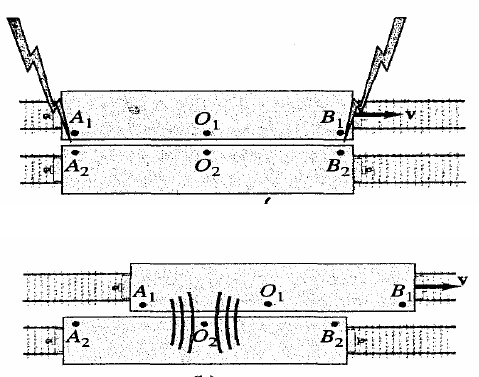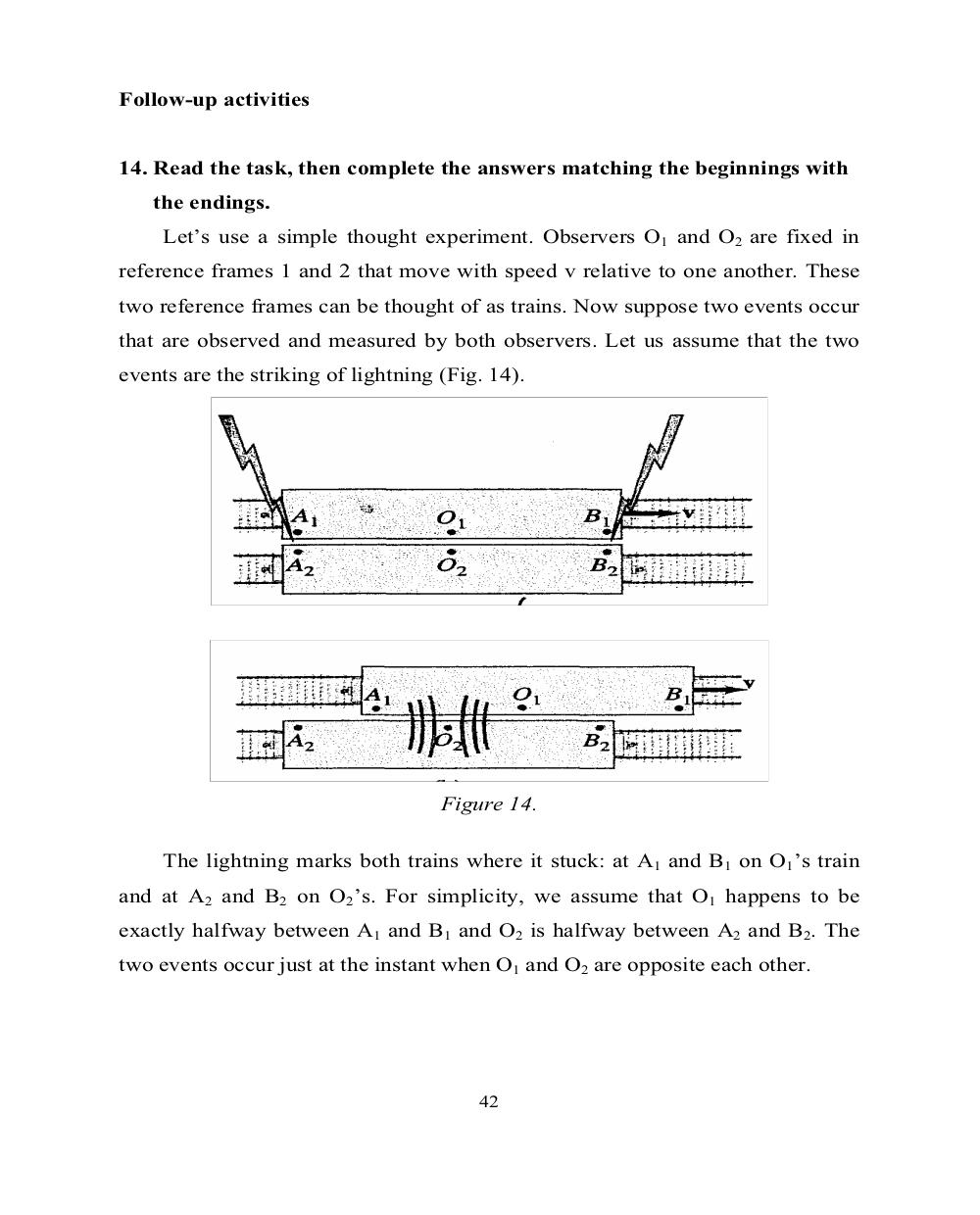# Английский язык. Ч.3 (Units 9,10). Ильичева Н.А - 42 стр.

Составители:

Рубрика:

• ## Иностранный языкFollow-up activities
14. Read the task, then complete the answers matching the beginnings with
the endings.
Let’s use a simple thought experiment. Observers O
1
and O
2
are fixed in
reference frames 1 and 2 that move with speed v relative to one another. These
two reference frames can be thought of as trains. Now suppose two events occur
that are observed and measured by both observers. Let us assume that the two
events are the striking of lightning (Fig. 14).
Figure 14.
The lightning marks both trains where it stuck: at A
1
and B
1
on O
1
’s train
and at A
2
and B
2
on O
2
’s. For simplicity, we assume that O
1
happens to be
exactly halfway between A
1
and B
1
and O
2
is halfway between A
2
and B
2
. The
two events occur just at the instant when O
1
and O
2
are opposite each other.
42Follow-up activities

14. Read the task, then complete the answers matching the beginnings with
the endings.
Let’s use a simple thought experiment. Observers O1 and O2 are fixed in
reference frames 1 and 2 that move with speed v relative to one another. These
two reference frames can be thought of as trains. Now suppose two events occur
that are observed and measured by both observers. Let us assume that the two
events are the striking of lightning (Fig. 14).

Figure 14.

The lightning marks both trains where it stuck: at A1 and B1 on O1’s train
and at A2 and B2 on O2’s. For simplicity, we assume that O1 happens to be
exactly halfway between A1 and B1 and O2 is halfway between A2 and B2. The
two events occur just at the instant when O1 and O2 are opposite each other.

42